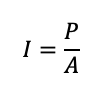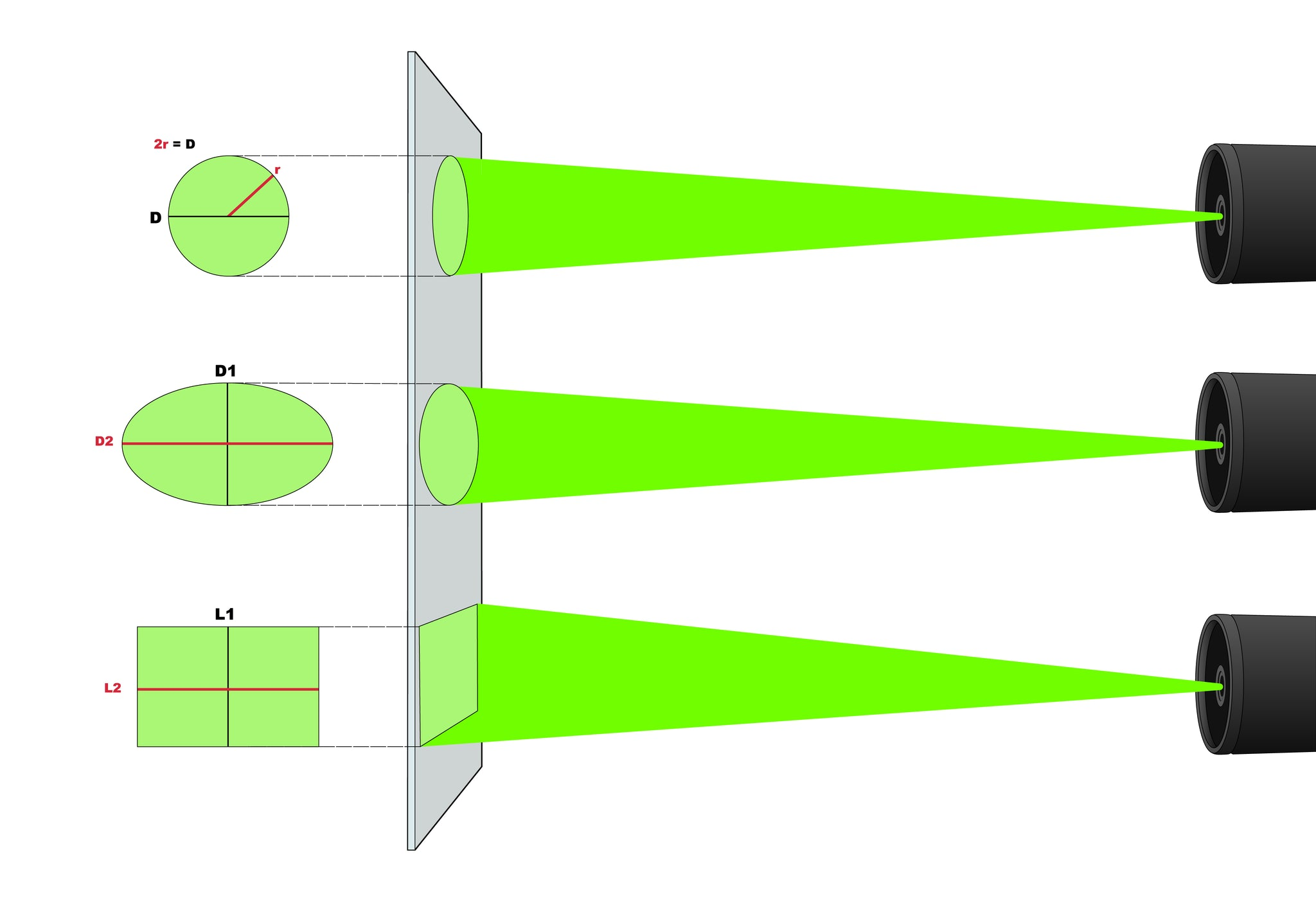In computing how much laser protection you needed, you ultimately need to determine the energy intensity (Power/Area or Energy/Area) and the exposure it needs to be reduced to for that wavelength - known as Maximum Permissible Exposure (MPE).Power or energy is known from the following:

• Power or energy of the beam
• Type of wavelength (CW, pulsed)

The area of the beam is the second component.  As the beams diameter becomes larger, it is important to know at what distance you are computing the area (or assume the worst-case).  For example, when purchasing a laser barrier, it is helpful to know how much protection is needed at a given point in the room.

Along with knowing the area, the intensity can vary at different points within the beam (or if a p-hat distribution, the intensity will be constant). This is important when calculating energy intensity as the area of the beam does not (if a gaussian distribution) have a constant intensity in the area of the beam.

To do so, the following parameters can be helpful:

• Shape of the beam
• Angle of divergence
• Distribution of the beam (Gaussian vs P-hat)
• Whether or not the beam is focused with a lens or not

The shape of the beam is part of the area calculation in the energy density equation. Below, we have depicted three different shapes and resultantly with a little geometry you will be able to see how the calculation of the area of the beam may change.The angle of divergence for the beam is also helpful if the laser is traveling over a long distance.  The image below illustrates how the diameter becomes larger over a greater distance. The rate at which this is happening is the angle of divergence.Another factor in calculation the energy density is understanding how the beams energy intensity is distributed. Most laser beams have a Gaussian distribution while others have what is known as a "p-hat" distribution - an equal level of intensity across the entire beam. The distribution has can have a significant impact when making laser safety calculations.

Please note that the image depicted below is 2-dimensional, while the distributions in reality are 3-dimensional space that take on the shape of the beam - in this case a circle. We've included an image of the surface area of a circular beam and the corresponding energy intensity of each distribution.